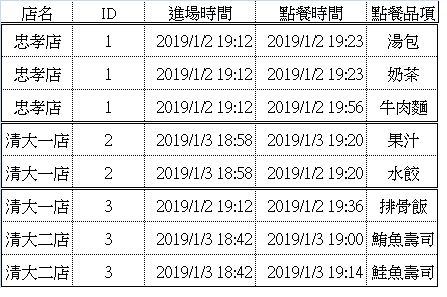#1

## R語言 rank公式

yaa <- y %>%
mutate(幾分鐘=difftime(點餐時間,進場時間,units = c("min")))%>%
group_by(店名,ID) %>%
rank(幾分鐘,na.last = TRUE, ties.method=c("min"))
Error in base::"rank"(x, ...) : unused argument (幾分鐘)listennn08 iT邦研究生 2 級 ‧ 2019-07-18 11:37:26 檢舉
mutate(幾分鐘,幾分鐘=difftime(點餐時間,進場時間,units = c("min")))%>%

### 1 個回答

0

rank只可以對一維度的向量(vector)進行排序。

``````yaa <- y %>%
mutate( 幾分鐘 = difftime( 點餐時間, 進場時間, units = c("min") ) ) %>%
arrange( 店名, ID, 幾分鐘 )
``````

``````yaa <- y %>%
mutate( 幾分鐘 = difftime( 點餐時間, 進場時間, units = c("min") ) ) %>%
group_by( 店名, ID ) %>%
distinct( 店名, ID, min(幾分鐘) )
``````

yaa <- y %>%
mutate( 幾分鐘 = difftime( 點餐時間, 進場時間, units = c("min") ) ) %>%
group_by( 店名, ID ) %>%
mutate(my_ranks = order(幾分鐘, decreasing=TRUE)) %>%
subset(my_ranks==1)

marlin12 iT邦新手 1 級 ‧ 2019-07-18 20:07:55 檢舉